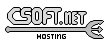Agar 1.7 Manual

M_Polygon(3)

# SYNOPSIS

```#include <agar/core.h>
#include <agar/gui.h>
#include <agar/math/m.h>
```

# DESCRIPTIONThe M_Polygon structure describes a simple polygon. The polygon may be convex or concave. Self-intersections and holes are not allowed.
```typedef struct m_polygon {
M_Vector2 *v;	/* Vertices */
Uint n;		/* Vertex count */
} M_Polygon;
```

# INITIALIZATION

void M_PolygonInit (M_Polygon *P)

M_Polygon M_POLYGON_INITIALIZER (void)

void M_PolygonFree (M_Polygon *P)

M_Polygon M_PolygonFromPts (Uint n, const M_Vector2 *v)

M_Polygon M_PolygonFromPointSet2 (const M_PointSet2 *ps)

M_Polygon M_PolygonFromPointSet2i (const M_PointSet2i *ps)

M_Polygon M_PolygonFromLines (Uint n, const M_Line2 *L)

M_PointSet2 M_PolygonToPointSet2 (const M_Polygon *P)

M_PointSet2i M_PolygonToPointSet2i (const M_Polygon *P, M_Real w, M_Real h)

void M_PolygonWrite (AG_DataSource *ds, const M_Polygon *P)

The M_PolygonInit() function initializes a M_Polygon structure. The M_POLYGON_INITIALIZER() macro may also be used as a static initializer.

The M_PolygonFree() function releases the memory allocated for vertices.

The M_PolygonFromPts() function returns a polygon structure given an array of points. The M_PolygonFromPointSet() variant accepts a M_PointSet2(3) argument. M_PolygonFromLines() returns a polygon from an array of lines L. n is the number of elements in the array.

The M_PolygonToPointSet2() and M_PolygonToPointSet2i() routines convert a M_Polygon to a M_PointSet2 or M_PointSet2i structure.

The M_PolygonRead() and M_PolygonWrite() functions read or write a polygon structure from/to an AG_DataSource(3).

# OPERATIONS

int M_PolygonAddVertex (M_Polygon *P, M_Vector2 v)

int M_PolygonAddLine (M_Polygon *P, M_Line2 L)

int M_PolygonDelVertex (M_Polygon *P, int v)

int M_PolygonCopy (M_Polygon *Pdst, const M_Polygon *Psrc)

void M_PolygonScale (M_Polygon *P, M_Real xScale, M_Real yScale)

void M_PolygonOffset (M_Polygon *P, M_Real xOffs, M_Real yOffs)

int M_PointInPolygon (const M_Polygon *P, M_Vector2 p)

int M_PolygonIsConvex (const M_Polygon *P)

The M_PolygonAddVertex() function adds a new vertex to a polygon. Returns index of new vertex on success, -1 on failure. The M_PolygonAddLine() variant accepts a M_Line(3) argument instead of a vector.

M_PolygonDelVertex() removes the vertex at given index v.

The M_PolygonCopy() function copies the vertices of a source polygon Psrc to a destination polygon Pdst. The destination structure does not need to be initialized. Any existing vertices in Pdst will be overwritten. The function returns 0 on success or -1 if insufficient memory is available.

M_PolygonScale() multiplies all vertices of a polygon with the given scaling factors xScale, yScale. M_PolygonOffset() translates all vertices against xOffs, yOffs.

The M_PointInPolygon() function returns 1 if the point p lies inside the polygon.

M_PolygonIsConvex() returns 1 if the polygon is convex.www.libAgar.org is © 2022 Julien Nadeau Carriere <vedge@csoft.net>.